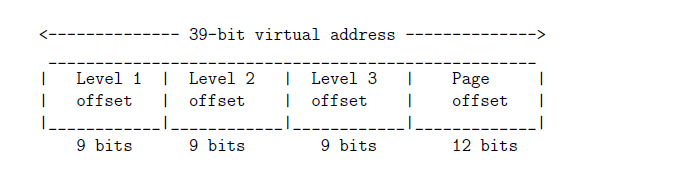# GATE | GATE CS 2021 | Set 2 | Question 58

• Last Updated : 23 May, 2021

Consider a three-level page table to translate a 39-bit virtual address to a physical address as shown below:The page size is 4 KB = (1KB = 210 bytes) and page table entry size at every level is 8 bytes. A process P is currently using 2 GB (1 GB = 230 bytes) virtual memory which OS mapped to 2 GB of physical memory. The minimum amount of memory required for the page table of P across all levels is _________ KB
(A) 4108
(B) 1027
(C) 3081
(D) 4698

Explanation: Given that Process P is using 2GB of physical memory and page size is 2^12 Bytes,

Number of pages = 2^31 / 2^12 = 2^19

Each page need a entry in 3rd level and there are 2^9 entries per page table in 3rd level.

So we need 2^19/2^9 = 2^10 page tables in 3rd level.

This implies there are 2^10 entries in 2nd level and there are 2^9 entries per page table in 2nd level.

So we need 2^10/2^9 = 2 page tables in 2nd level.

Now we have 2 entries in 1st level and hence, we need only 1 page table in 1st level.

So on total we need,
2^10 +2 +1 = 1027 page tables each with 2^9 entries of size 8 bytes.

Total size of page tables = 1027*2^9*8 Bytes

= 1027*2^12 Bytes
= 1027*4 KB
= 4108 KB

Quiz of this Question

My Personal Notes arrow_drop_up
Recommended Articles
Page :